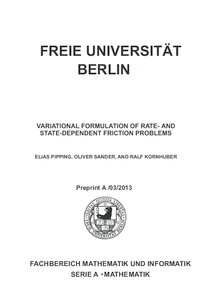Repository: Freie Universität Berlin, Math Department

# Variational formulation of rate- and state-dependent friction problems

Pipping, E. and Sander, O. and Kornhuber, R. (2015) Variational formulation of rate- and state-dependent friction problems. Zeitschrift für angewandte Mathematik und Mechanik (ZAMM), 95 (4). pp. 377-395. ISSN 0044-2267Preview

872kB

Official URL: http://dx.doi.org/10.1002/zamm.201300062

## Abstract

We propose a variational formulation of rate- and state-dependent models for the dynamic sliding of a linearly elastic block on a rigid surface in terms of two coupled variational inequalities. Classical Dieterich–Ruina models are covered as special cases. We show existence and uniqueness of solutions for the two spatial subproblems arising from time discretisation. Existence of solutions to the coupled spatial problems is established for Dieterich's state equation through a fixed point argument. We conclude with some numerical experiments that suggest mesh independent convergence of the underlying fixed point iteration, and illustrate quasiperiodic occurrence of stick/slip events.

Item Type: Article Continuum mechanics, frictional contact problems, gradient flows, finite elements Mathematical and Computer Sciences > Mathematics > Numerical Analysis Department of Mathematics and Computer Science > Institute of Mathematics 1783 Ekaterina Engel 17 Feb 2016 08:33 03 Mar 2017 14:41

Repository Staff Only: item control page